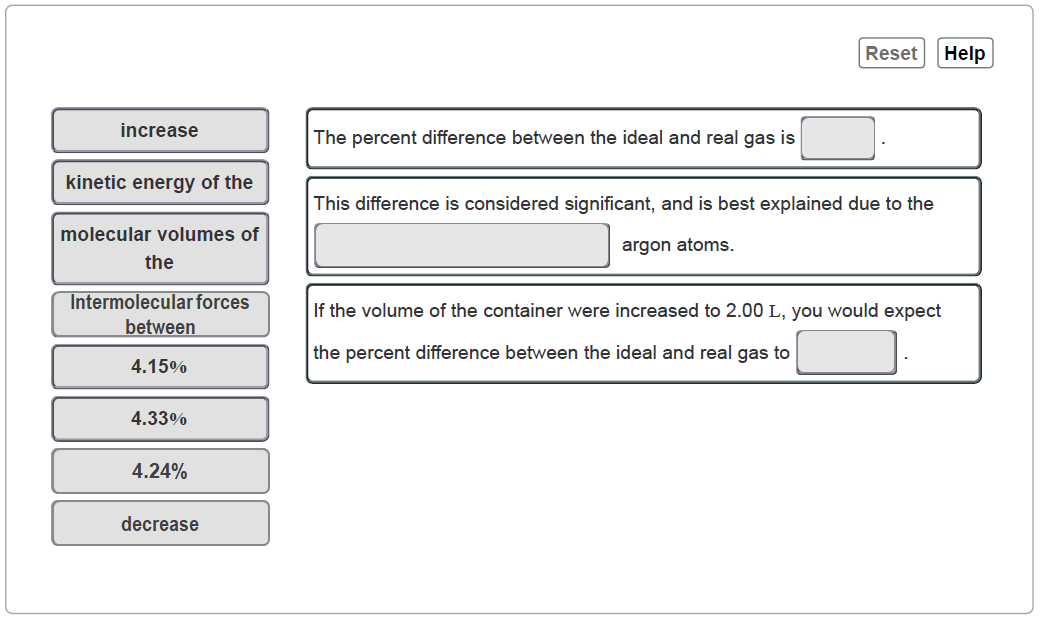# Problem: In Part B the given conditions were 1.00 mol of argon in a 0.500-L container at 24.0 °C. You identified that the ideal pressure (Pideal) was 48.8 atm, and the real pressure (Preal) was 46.7 atm under these conditions. Complete the sentences to analyze this difference.Match the words and compounds in the left column to the appropriate blanks in the sentences on the right. Make certain each sentence is complete before submitting your answer.Ideal versus real behavior for gasesIn the following part you can see how the behavior of real gases deviates from the ideal behavior. You will calculate the pressure values for a gas using the ideal gas law and also the van der Waals equation. Take note of how they differ.

###### FREE Expert Solution
79% (377 ratings)View Complete Written Solution
###### Problem Details

In Part B the given conditions were 1.00 mol of argon in a 0.500-L container at 24.0 °C. You identified that the ideal pressure (Pideal) was 48.8 atm, and the real pressure (Preal) was 46.7 atm under these conditions. Complete the sentences to analyze this difference.

Match the words and compounds in the left column to the appropriate blanks in the sentences on the right. Make certain each sentence is complete before submitting your answer.Ideal versus real behavior for gases

In the following part you can see how the behavior of real gases deviates from the ideal behavior. You will calculate the pressure values for a gas using the ideal gas law and also the van der Waals equation. Take note of how they differ.